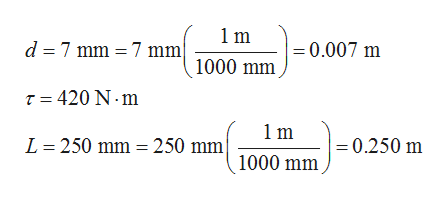# The  7 mm bolt head shown must be tightened to a torque of 420 N-m. Determine the (a) force (N) that delivers this torque if it is applied perpendicular to wrench a distance L = 250 mm.   (b) Assume the wrench has a hexagonal box end and determine the local tangential force (N) applied to each of the six contact points of the box wrench.   (c) Now assume the wrench has an 'open end' as shown below, and determine the local tangential force (N) applied to each of the two contact points.

Question
88 views
 The  7 mm bolt head shown must be tightened to a torque of 420 N-m. Determine the (a) force (N) that delivers this torque if it is applied perpendicular to wrench a distance L = 250 mm.   (b) Assume the wrench has a hexagonal box end and determine the local tangential force (N) applied to each of the six contact points of the box wrench.   (c) Now assume the wrench has an 'open end' as shown below, and determine the local tangential force (N) applied to each of the two contact points.
check_circle

Step 1

Consider the diameter of the bolt be d, the torque applied to the bolt be τ, and the perpendicular distance of the applied force from the center of the bolt be L.

The given values are,help_outlineImage Transcriptionclose1m d 7 mm 7 mm =0.007 m 1000 mm T 420 N m 1m L 250 mm 250 mm =0.250 m 1000 mm fullscreen
Step 2

(a)

Write the expression for the torque by a force, and solve for the force applied to the bolt at a perpendicular distance L.

Step 3

(b)

The free-body diagram of the head...

### Want to see the full answer?

See Solution

#### Want to see this answer and more?

Solutions are written by subject experts who are available 24/7. Questions are typically answered within 1 hour.*

See Solution
*Response times may vary by subject and question.
Tagged in

### Newtons Laws of Motion